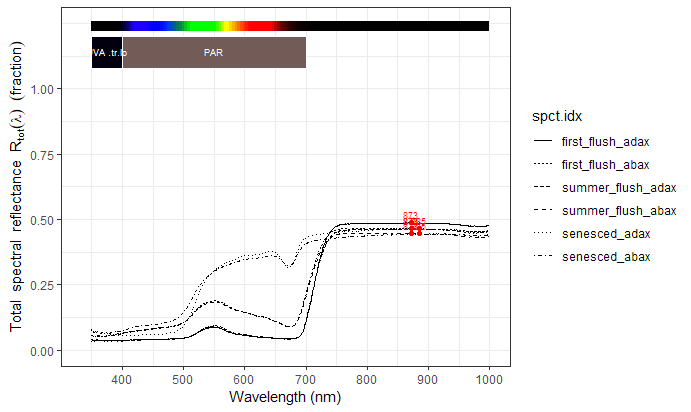# User Guide

## Introduction

This package provides data sets and functions relevant to the photobiology of plants. It is part of a suite, which has package ‘photobiology’ at its core. Please visit (http://www.r4photobiology.info/) for more details. For more details on plotting spectra, please consult the documentation for package ‘ggspectra’, and for information on the calculation of summaries and maths operations between spectra, please, consult the documentation for package ‘photobiology’.

See the User Guide for package ‘photobiology’ for instructions on how to work with spectral data.

library(ggspectra)
library(photobiology)
library(photobiologyPlants)
library(photobiologyWavebands)

theme_set(theme_bw())

## Photon ratios relevant to plants

R_FR(), B_G(), UVB_UV(), UVA_UV(), UV_PAR(), UVB_PAR(), UVA_PAR() are convenience functions implemented using function q_ratio() from package ‘photobiology’ and waveband definitions from package ‘photobiiologyWavebands’ with defaults as commonly used in the field of plant photobiology.

R_FR(sun.spct)
##  Red.Smith10: FarRed.Smith10(q:q)
##                          1.266704
## attr(,"radiation.unit")
##  "q:q ratio"

## Calculating the phytochrome photoequilibrium

In the examples below we use the solar spectral data included in package ‘photobiology’ as a data frame in object sun.spct.

We can calculate the phytochrome photoequilibrium from spectral irradiance data contained in a source_spct object as follows.

Pfr_Ptot(sun.spct)
##  0.68341

We can also calcualte the red:far-red photon ratio, in this second example, for the same spectrum as above

R_FR(sun.spct)
##  Red.Smith10: FarRed.Smith10(q:q)
##                          1.266704
## attr(,"radiation.unit")
##  "q:q ratio"

which is equivalent to calculating it using package ‘photobiology’ directly

q_ratio(sun.spct, Red("Smith10"), Far_red("Smith10"))
##  Red.Smith10: FarRed.Smith10(q:q)
##                          1.266704
## attr(,"radiation.unit")
##  "q:q ratio"

We can, and should whenever spectral data are available, calculate the photoequilibrium as above, directly from these data. It is possible to obtain and approximation in case of the solar spectrum and other broad spectra, using the red:far-red photon ratio. The calculation, however, is only strictly valid, for di-chromatic illumination with red plus far-red light.

Pfr_Ptot_R_FR(R_FR(sun.spct))
##  Red.Smith10: FarRed.Smith10(q:q)
##                         0.7051691

Here we calculated the R:FR ratio from spectral data, but in practice one would use this function only when spectral data is not available as when a R plus FR sensor is used. We can see that in such a case the photoequilibrium calculated is only a rough approximation. For sunlight, in the example above when using spectral data we obtained a value of 0.683 in contrast to 0.705 when using the R:FR photon ratio. For other light sources differences can be much larger. Furthermore, here we used the true R:FR ratio calculated from the spectrum, while broad-band red:far-red sensors guive only an approximation, which is good for sunlight, but which will be innacurate for artifical light, unless a special calibration is done for each type of lamp.

In the case of monochromatic light we can still use the same functions, as the defaults are such that we can use a single value as the ‘w.length’ argument, to obtain the Pfr:P ratio. For monochromatic light, irradiance is irrelevant for the photoequilibrium (steady-state).

Pfr_Ptot(660)
##  0.869649
Pfr_Ptot(735)
##  0.01749967
Pfr_Ptot(c(660, 735))
##  0.86964902 0.01749967
Pfr_Ptot(435)
##  0.3859998

We can also plot Pfr:Ptot as a function of wavelength (nm) of monochromatic light. The default is to return a vector for short input vectors, and a response_spct object otherwise, but this can be changed through argument spct.out.

autoplot(Pfr_Ptot(300:770), norm = NULL, unit.out = "photon",
w.band = Plant_bands(),
annotations = c("colour.guide", "labels", "boxes")) +
labs(y = "Phytochrome photoequilibrium, Pfr:Ptot ratio")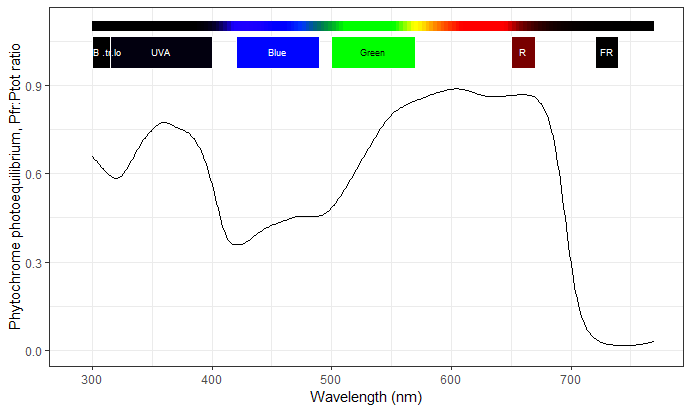It is, of course, also possible to use base R plotting functions, or as shown here functions from package ‘ggplot2’

ggplot(data = Pfr_Ptot(300:770), aes(w.length, s.q.response)) +
geom_line() +
labs(x = "Wavelength (nm)",
y = "Phytochrome photoequilibrium, Pfr:Ptot ratio")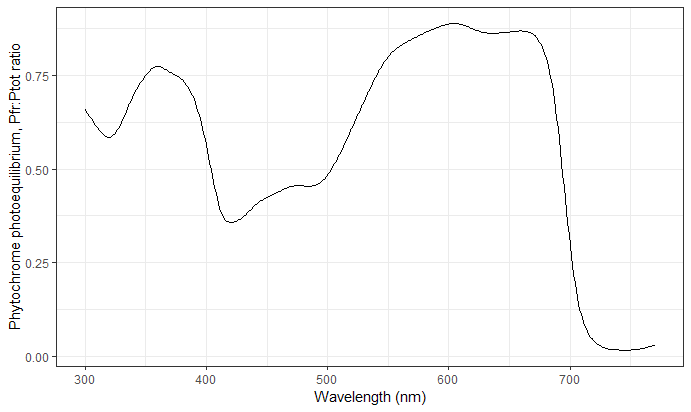In the case of dichromatic illumination with red (660 nm) and far-red (730 nm) light, we can use a different function that takes the R:FR photon ratio as argument.

These computations are valid only for true mixes of light at these two wavelengths but not valid for broad spectra like sunlight and especially inaccurate for plant growth lamps with peaks in their output spectrum, such as most discharge lamps (sodium, mercury, multi-metal, fluorescent tubes) and many LED lamps.

Pfr_Ptot_R_FR(1.15)
##  0.6919699
Pfr_Ptot_R_FR(0.01)
##  0.04747996
Pfr_Ptot_R_FR(c(1.15,0.01))
##  0.69196990 0.04747996

It is also easy to plot Pfr:P ratio as a function of R:FR photon ratio. However we have to remember that such values are exact only for dichromatic light, and only a very rough approximation for wide-spectrum light sources. For wide-spectrum light sources, the photoequilibrium should, if possible, be calculated from spectral irradiance data.

ex6.data <- data.frame(r.fr=seq(0.01, 5.0, length.out=100), Pfr.p=numeric(100))
ex6.data$Pfr.p <- Pfr_Ptot_R_FR(ex6.data$r.fr)
ggplot(data=ex6.data, aes(r.fr, Pfr.p)) +
geom_line() +
labs(x ="R:FR photon ratio",
y = "Phytochrome photoequilibrium, Pfr:Ptot ratio")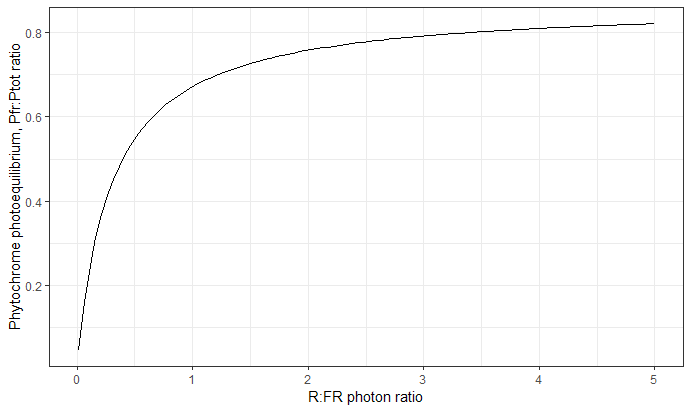## Calculating reaction rates

with(clip_wl(sun.spct, c(300,770)), Phy_reaction_rates(w.length, s.e.irrad))
## $k1 ##  1.259332 ## ##$k2
##  0.5833862
##
## $nu ##  1.842718 ## Calculating the absorption cross section at given wavelengths The phytochrome photoequilibrium cannot be calculated from the absorptance spectra of Pr and Pfr, because Pr and Pfr have different quantum yields for the respective phototransformations. We need to use action spectra, which in this context are usually called absorption cross-sections'. They can be calculated as the product of absorptance and quantum yield. The values in these spectra, in the case of Phy are calledSigma’. Here we reproduce Figure 3 in Mancinelli (1994), which gives the ‘Relative photoconversion cross-sections’ of Pr ($$\sigma_R$$) and Pfr ($$\sigma_{FR}$$). The values are expressed relative to $$\sigma_R$$ at its maximum at $$\lambda = 666$$ nm. ex7.data <- data.frame(w.length=seq(300, 770, length.out=100)) ex7.data$sigma.r <- Phy_Sigma_R(ex7.data$w.length) ex7.data$sigma.fr <- Phy_Sigma_FR(ex7.data$w.length) ex7.data$sigma <- Phy_Sigma(ex7.data$w.length) ggplot(ex7.data, aes(x = w.length)) + geom_line(aes(y = sigma.r/ max(sigma.r)), colour = "red") + geom_line(aes(y = sigma.fr/ max(sigma.r))) + labs(x = "Wavelength (nm)", y = expression(sigma[R]~"and"~sigma[FR]))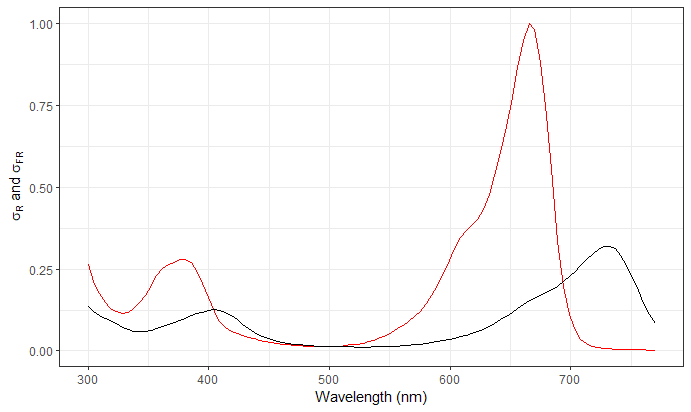rm(ex7.data) ## Spectral absorbance of cryptochromes names(CRYs.mspct) ##  "CRY1_dark" "CRY1_light" "CRY2_dark" "CRY2_light" "CRY3_dark" Here we approximate Figure 1.B from Banerjee et al. (2007). A_as_default() autoplot(interpolate_wl(CRYs.mspct$CRY2_dark, 300:500))
## Warning: Removed 10 rows containing non-finite values (stat_peaks).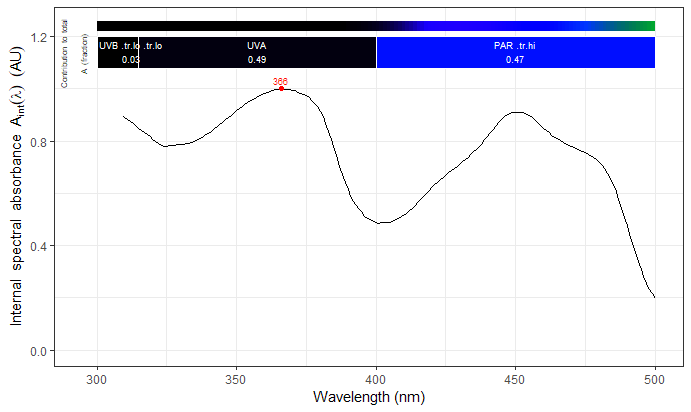autoplot(CRYs.mspct[c("CRY2_dark", "CRY2_light")], range = c(300,700))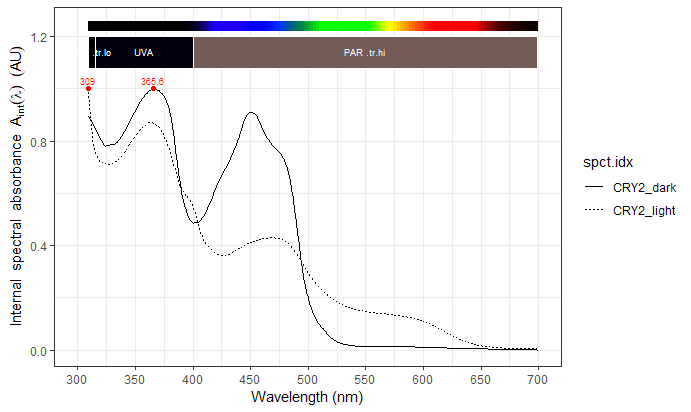autoplot(CRYs.mspct[c("CRY1_dark", "CRY1_light")])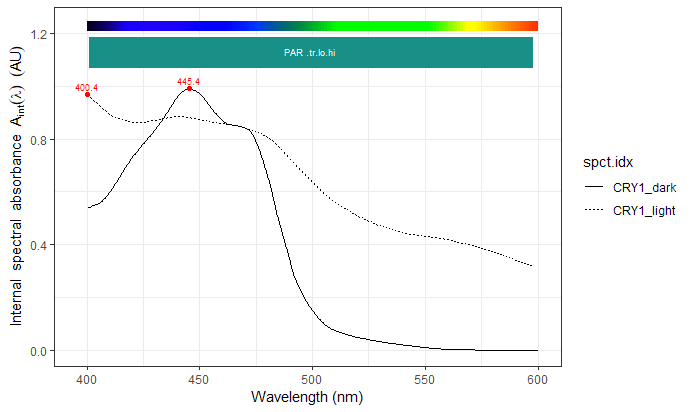autoplot(CRYs.mspct["CRY3_dark"], range = c(300,700))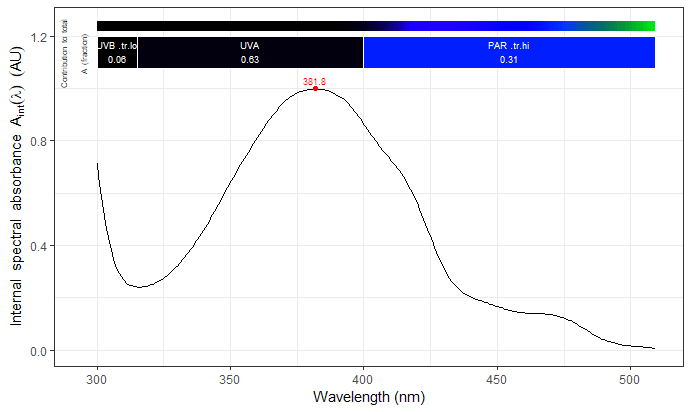ggplot(CRYs.mspct[c("CRY1_dark", "CRY2_dark", "CRY3_dark")]) +
geom_line(aes(linetype = spct.idx)) +
expand_limits(x = 300)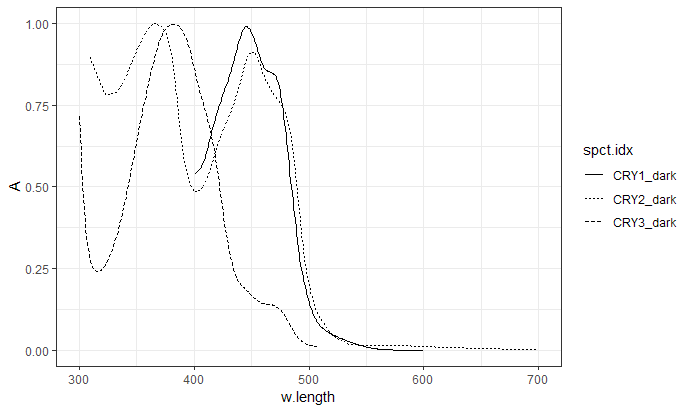## Spectral absorbance of phototropins

names(PHOTs.mspct)
##  "PHOT1_fluo"  "PHOT2_fluo"  "PHOT1_dark"  "PHOT1_light"
autoplot(PHOTs.mspct[c("PHOT1_fluo", "PHOT2_fluo")]) +
expand_limits(x = 300)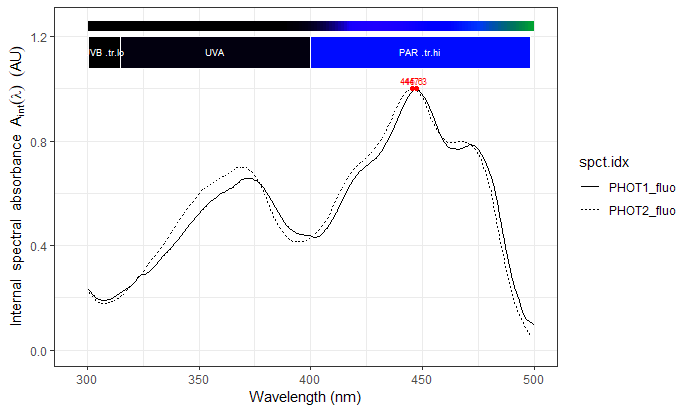autoplot(PHOTs.mspct[c("PHOT1_dark", "PHOT1_light")])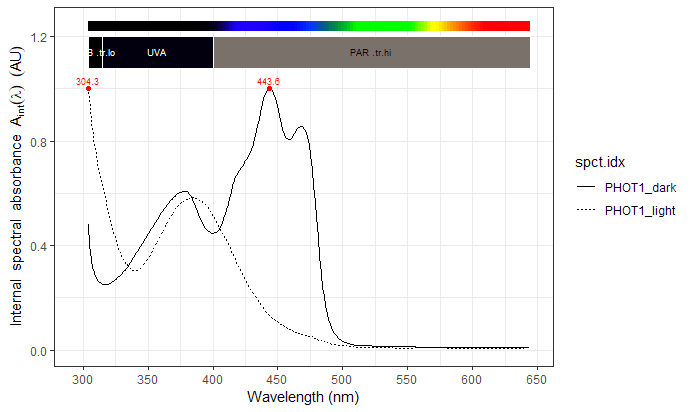## UV8 absorbance

autoplot(UVR8s.mspct)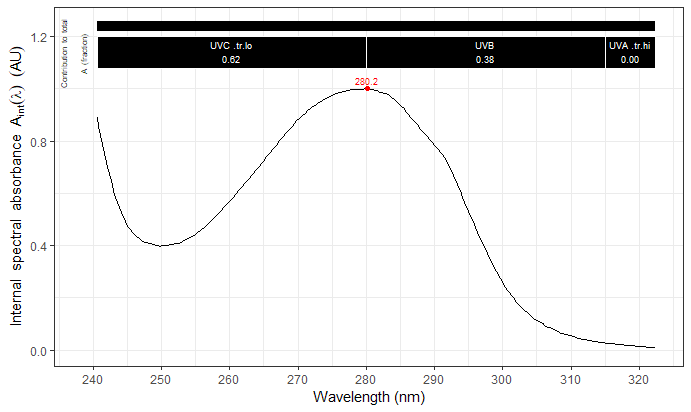## Spectral absorbance of zeitloupe proteins

names(ZTLs.mspct)
##  "ZTL_dark"  "ZTL_light"
autoplot(ZTLs.mspct) +
expand_limits(x = 300)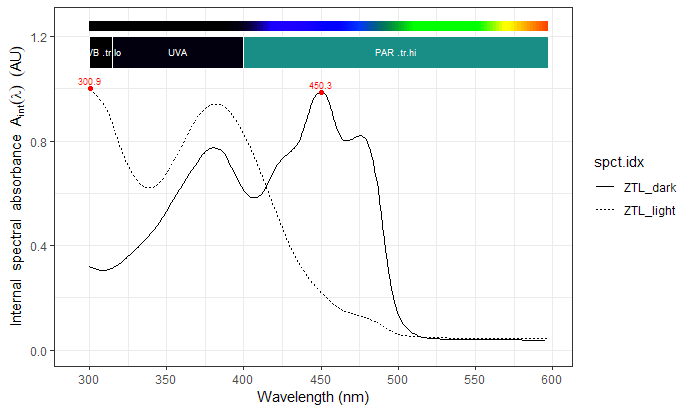## Photosynthesis action spectra

photon_as_default()
names(McCree_photosynthesis.mspct)
##  "amaranth" "oats"
autoplot(McCree_photosynthesis.mspct)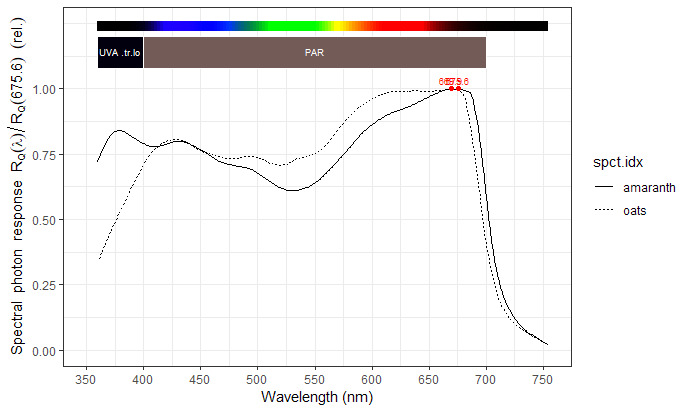## Carotenoids

A_as_default()
names(carotenoids.mspct)
##  "beta_carotene"    "dihydro_lycopene" "lycopene"
##  "lutein"           "phytoene"         "phytofluene"
##  "violaxanthin"     "zeaxanthin"
autoplot(carotenoids.mspct[1:4],
annotations = c("-", "labels", "boxes"))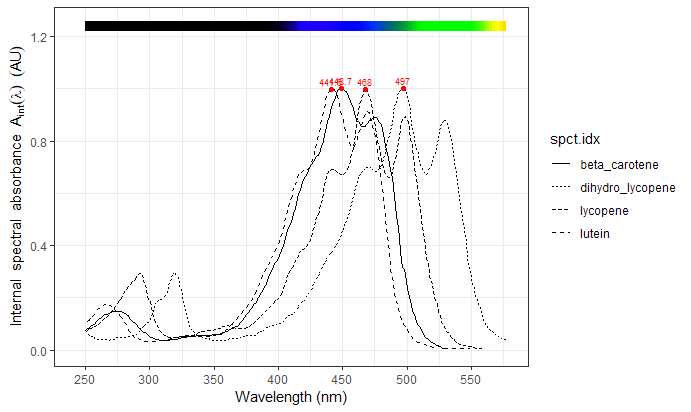autoplot(carotenoids.mspct[5:length(carotenoids.mspct)],
annotations = c("-", "labels", "boxes"))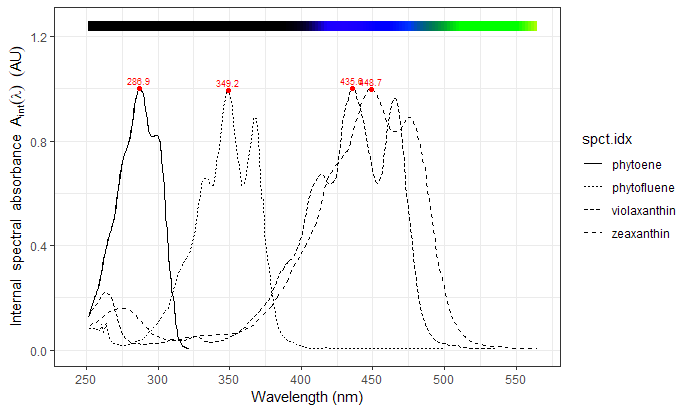## Chlorophylls

A_as_default()
names(chlorophylls.mspct)
##  "Chl_a_MethOH" "Chl_a_DME"    "Chl_b_DME"
autoplot(chlorophylls.mspct[c("Chl_a_DME", "Chl_b_DME")])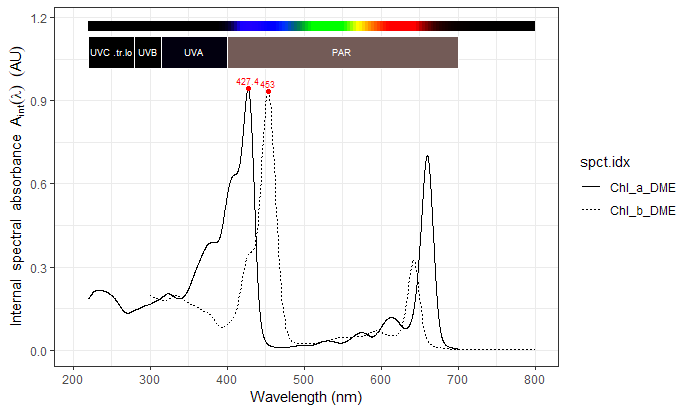autoplot(chlorophylls.mspct[c("Chl_a_DME", "Chl_a_MethOH")])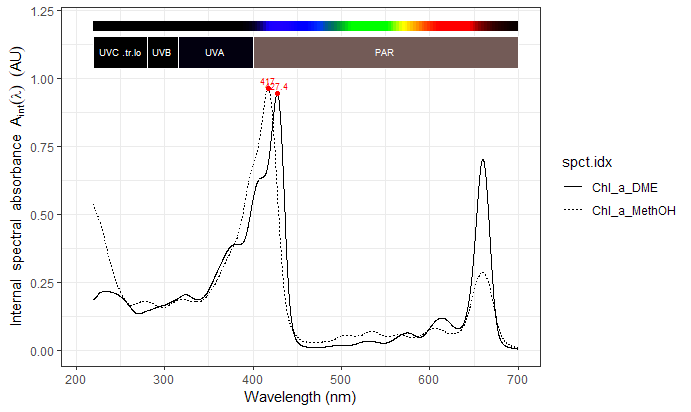### Fluorescence

names(chlorophylls_fluorescence.mspct)
##  "Chl_a_MethOH" "Chl_a_DME"    "Chl_b_DME"
autoplot(chlorophylls_fluorescence.mspct[c("Chl_a_DME", "Chl_b_DME")]) 
## Warning: Removed 67 rows containing non-finite values (stat_peaks).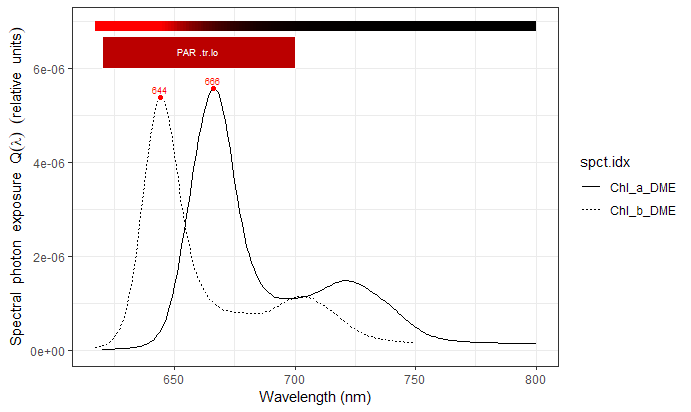autoplot(chlorophylls_fluorescence.mspct[c("Chl_a_DME", "Chl_a_MethOH")]) 
## Warning: Removed 16 rows containing non-finite values (stat_peaks).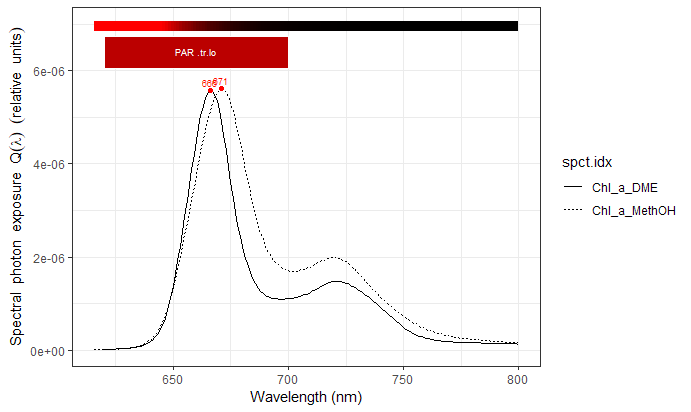## Optical properties of leaves

Tfr_as_default()
names(Solidago_altissima.mspct)
##  "lower_adax" "lower_abax" "upper_adax" "upper_abax"
autoplot(Solidago_altissima.mspct$lower_adax)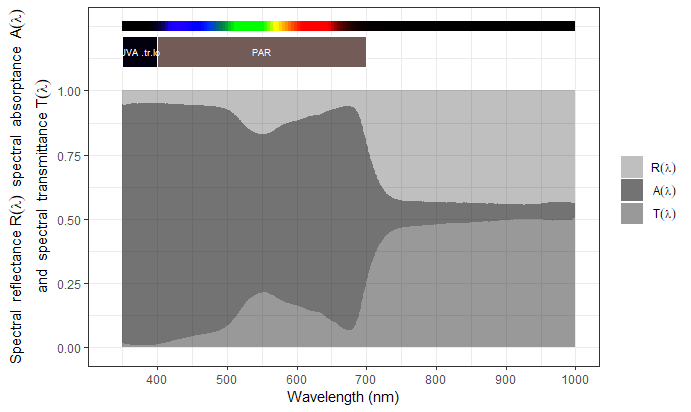autoplot(Solidago_altissima.mspct$lower_abax)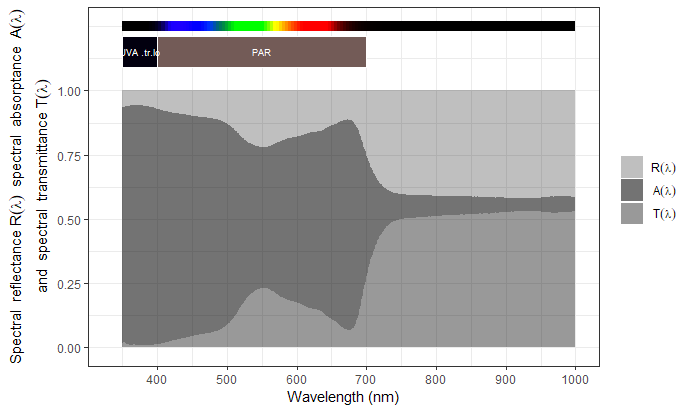autoplot(as.filter_mspct(Betula_ermanii.mspct))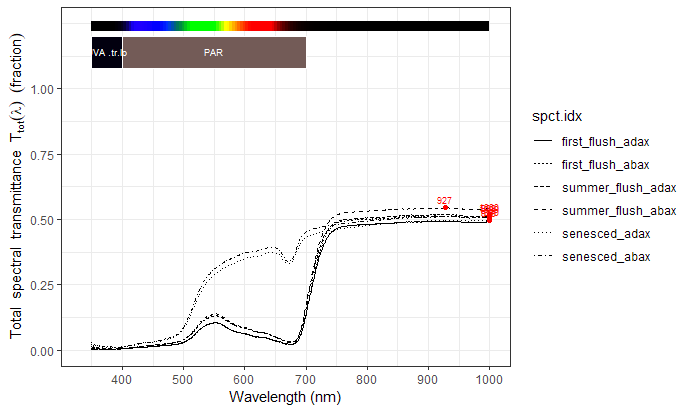autoplot(as.reflector_mspct(Betula_ermanii.mspct))